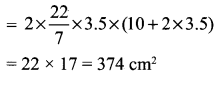You can Download Samacheer Kalvi 10th Maths Book Solutions Guide Pdf, Tamilnadu State Board help you to revise the complete Syllabus and score more marks in your examinations.

## Tamilnadu Samacheer Kalvi 10th Maths Solutions Chapter 7 Mensuration Additional Questions

Question 1.
If the radii of the circular ends of a conical bucket which is 45 cm high are 28 cm and 7 cm, find the capacity of the bucket. (Use $$\pi=\frac{22}{7}$$)
Solution:
Clearly bucket forms frustum of a cone such that the radii of its circular ends are r1 = 28 cm, r2 = 7 cm, h = 45 cm.
Capacity of the bucket = volume of the frustum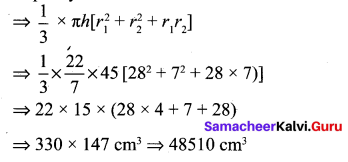Question 2.
Find the depth of a cylindrical tank of radius 28 m, if its capacity is equal to that of a rectangular tank of size 28 m × 16 m × 11 m.
Solution:
Volume of the cylindrical tank = Volume of the rectangle tank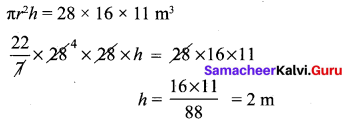Question 3.
What is the ratio of the volume of a cylinder, a cone, and a sphere. If each has the same diameter and same height?
Solution: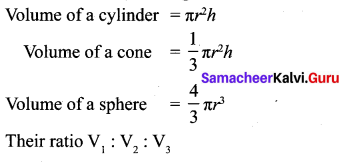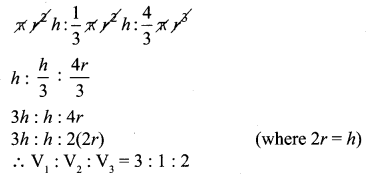Question 4.
Find the number of coins, 1.5 cm is diameter and 0.2 cm thick, to be melted to form a right circular cylinder of height 10 cm and diameter 4.5 cm.
Solution: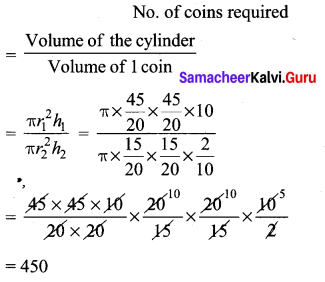Question 5.
A spherical ball of iron has been melted and made into small balls. If the raidus of each smaller ball is one-fourth of the radius of the original one, how many such balls can be made?
Solution: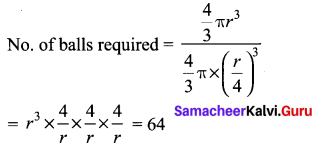Question 6.
A wooden article was made by scooping out a hemisphere from each end of a cylinder as shown in figure. If the height of the cylinder is 10cm and its base is of radius 3.5 cm find the total surface area of the article.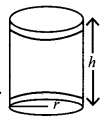Solution:
Radius of the cylinder be r Height of the cylinder be h Total surface area of the article = CSA of cylinder + CSA of 2 hemispheres = 2πrh + 2πr2 = 2πr (h + 2r)